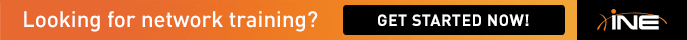# Math on the QoS Section

Can any one explain how the math worked out in the solution guide?

I can't make any sense of it.

• 8.3: Burst in bytes = (Police Rate in bits / X) / 8 bits in each byte

X = Intervals per second = 1000 msec / 125 msec = 8

8.4: Burst in bytes = (Police Rate in bits / X) / 8 bits in each byte

X = Intervals per second = 1000 msec / 200 msec = 5

For example, the busrt for the SILVER class is... Burst = (3500000 / 5) / 8 = 87500